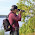## Monday, January 14, 2013

### NFL Picks Contest - Results and Updated Standings

Here are the results and updated standings in the NFL Picks Contest.

Vegas Vic, who won the first NFL Picks Contest earlier this season, takes the lead from MOJO with only three games left from which to pick.

lightning- New England (W 41-28) = 23 pts.
TwoMinuteWarning- New England (W 41-28) = 23 pts.
Grange95-  Denver (L-35-38) = <3> pts.
Music City Momma- New England (W 41-28) = 23 pts.
Memphis MOJO-  Denver (L 35-38) = <3> pts.
ggrouchie- No Pick = 0 pts.
Vegas Vic-  New England (W 41-28) = 23 pts.
Captain Crunch- New England (W 41-28) = 23 pts.

Here are the current standings;

Vegas Vic - 16+19+22+13+16+40+15+23+43+58+24+24+23 = 336
Memphis MOJO - 40+19+22+13+16+18+24+68+22+17+45+24-(3) = 325 pts.
TwoMinuteWarning - 13+19+22+16+31+18+19+23+31+58+24+24+23 =321
Music City Momma - 17+19+24+5+17+34+24+24+43+17+24+24+23 = 295
Grange95 - 20+19+22+13+14+40+24+23+43+17+45-(15)-(3) = 262
lightning - 13+19+24+13+16+18+24+24+22+17+38+24+23 =275
Captain Crunch - 13+11+18+16+31+18+24-(2)+31+32+24+20+23 =259
grrouchie - 14+48+20+5+16+40+17+23-(28)+17+24+25+0 = 221

Good luck in the conference championship games.

#### 1 comment:

1.Denver, arrgh.